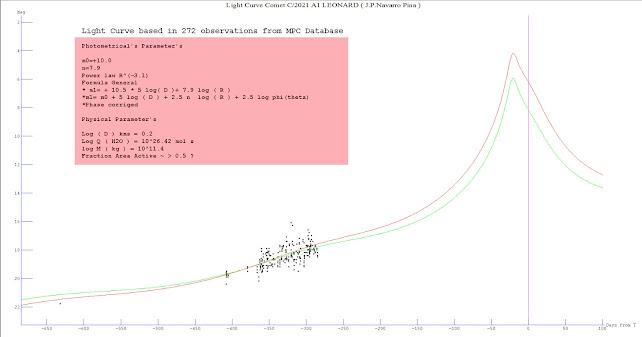## viernes, 23 de octubre de 2015

### ANALYSIS OF THE LIGHT VISUAL AND CCD CURVE OF COMET C/2013 US10 CATALINA

Hi all , based in the analysis of the light visual and ccd curve of comet C/2013 US10 CATALINA and recopilation from database MPC /IAU and list of comet obs and observadorescometas yahoogroups.com of 1.210 observations , i analyzed the graphic and data and obtained than the probability of visual maximum magnitude for this comet is m1(max)~ 6.0 for 2016 , my differential analysis of the light curve based on a sum of observations CCDs and other visual and visual only gives the result for only next formula : m1=5.4+5log(d)+7.8log(r) and the poor activity index n=3.1 this result indicate than brightness of comet is a comet that behaves solid object type asteroid in actually heliocentric distance R=1.00 au , this is very typical of the new comets Oort Cloud (e= 1.000322) when they exceed the heliocentric distance of r = 1.5 au brightness stagnation and stifling dust activity and especially the gas is produced, in my opinion this activity remains off and could be reactivated in February 2016. ** Updated ( 25/10/2015 ) 1 .-The new calculations of J.P.Navarro based in the reference of A.Sosa and J.A. Fdez. from ''Masses of long period comets'' ( 2011 ) , show new physical parameters of comet C/2013 US10 CATALINA : The Log10 ( water production rate ) maximum based in correlation between visual total heliocentric magnitudes for US10 CATALINA show 10^29,5 MOLEC/S ( ~ 3.000 kg / sec )in R = 1.0 au . 2.-The Fraction active surface area calculated by Navarro Pina is FASA=2.4 , for comparison the comet C/2004 Q2 is FASA=2.4 , C/2007 W1 FASA=2.3 , this indicate high rate of fraction surface area for US10 CATALINA , by example the 1P/Halley FASA=0.2 . 3.-The Mass estimated is Log10 M (kg) =13.00 based in absolute magnitude for total visuals observations m0=5.4 , 10^12 kg . 4.-The Diameter calculated is log10(D)=0.6 kms. , based in the formula log10 D (km)=1.5-0.13*m0 ( Sosa & Fdez 2011 )### Analysis Light Curve COMET 2021 A1 LEONARD

Comet C / 2021 A1 Leonard shows at the moment with 272 ccd observations a linear fit in its light curve, the provisional photometric parame...